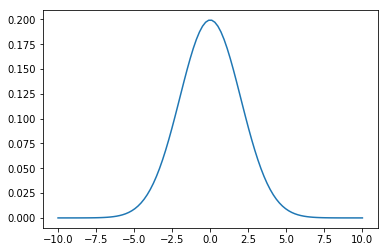# Plotting Graphs¶

In this notebook, we will cover variables in Python, Numpy arrays, and plotting with Matplotlib.

We will mostly be following Chapter 1 of Programming for Computations - Python by Svein Linge and Hans Petter Langtangen.

## Variables¶

Variables can be assigned values with the = operator.

In :
x = 3


The variable keeps the same value until it is changed by the program.

In :
print(x)

3


In math the statement x=x+1 is always False (assuming x is a real number). In Python, it means that the program should first compute the value of x+1 and then store it in the variable x. This results in 4 being stored in x.

In :
x = x+1
print(x)

4


Note that variables can store more than just numbers. Here, we store and add two strings (which concatenates).

In :
s1 = "We are using "
s2 = "Python to compute."
print(s1+s2)

We are using Python to compute.


Here we store a 4-tuple in v.

In :
v = (1,2,3,4)
print(v)

(1, 2, 3, 4)


Tuples are not quite vectors, for example, 3*v is three copies of v concatenated.

In :
3*v

Out:
(1, 2, 3, 4, 1, 2, 3, 4, 1, 2, 3, 4)

In :
v + (5,6)

Out:
(1, 2, 3, 4, 5, 6)

You can access the individual entries using the form v[i] where i runs from zero up through one less than the number of entries in v. For example the 4th entry can be obtained by:

In :
v

Out:
4

We'll come back to discussing tuples later in the course.

## Numerical Python (NumPy)¶

Numpy is the main numerical math package. It has support for vectors and matrices that Python lacks.

You load Numpy with the command:

In :
import numpy as np    # standard way of importing numpy


Now commands in the Numpy package can be called with the syntax np.command.

(The text after # above is a comment and is ignored by Python.)

In lieu of tuples, we can use numpy arrays for vectors. For example:

In :
v = np.array([1,2,3,4])
v

Out:
array([1, 2, 3, 4])
In :
3*v

Out:
array([ 3,  6,  9, 12])
In :
v+v

Out:
array([2, 4, 6, 8])

Computing cos(v) with cos from the math package will fail, but numpy has its own cosine function which evaluates termwise. So, np.cos(v) will compute $$(\cos 1, \cos 2, \cos 3, \cos 4).$$

In :
np.cos(v)

Out:
array([ 0.54030231, -0.41614684, -0.9899925 , -0.65364362])

You can type np. and press the Tab key to see what commands are available. Then you can type np.abs? and Shift-Enter (for example) to see the documentation of a command.

## Plotting with Matplotlib¶

You can import Mathplotlib's pyplot using:

In :
import matplotlib.pyplot as plt

In :
x = np.array([-2, -1, 0, 1, 2])


We'll plot the points $(x_i,y_i)$ where $x_i$ is in the list above and $y_i=x_i^2-1$. Arithmetic in arrays is done component wise. So the following list gives the y-values:

In :
y = x*x-1
y

Out:
array([ 3,  0, -1,  0,  3])

The following gives a plot of the points $(x_i, y_i)$:

In :
plt.plot(x, y, 'ro')
plt.show()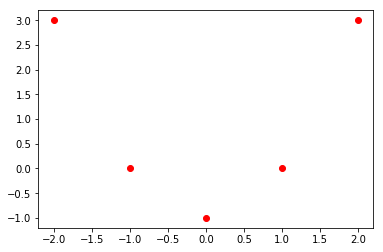The string 'ro' above indicates that this is a list plot: The o indicates to plot points, and the r indicates to plot in red. Other options for plotting can be found by typing plt.plot? and Shift+Enter. You need to scroll down to the bottom of the Notes section to see a complete description of the fmt string. Alternately take a look at the api posted to the matplotlib website.

In :
plt.show(plt.plot(x, y))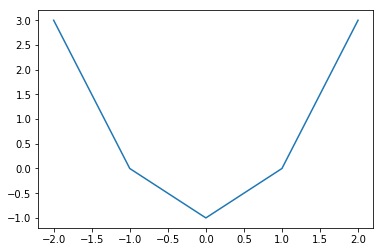To get a nicer curve, we should use more points. The following gives 101 points equally spaced between -2 and 2:

In :
x = np.linspace(-2, 2, 101) # 1001 might be better than 101...
x

Out:
array([-2.  , -1.96, -1.92, -1.88, -1.84, -1.8 , -1.76, -1.72, -1.68,
-1.64, -1.6 , -1.56, -1.52, -1.48, -1.44, -1.4 , -1.36, -1.32,
-1.28, -1.24, -1.2 , -1.16, -1.12, -1.08, -1.04, -1.  , -0.96,
-0.92, -0.88, -0.84, -0.8 , -0.76, -0.72, -0.68, -0.64, -0.6 ,
-0.56, -0.52, -0.48, -0.44, -0.4 , -0.36, -0.32, -0.28, -0.24,
-0.2 , -0.16, -0.12, -0.08, -0.04,  0.  ,  0.04,  0.08,  0.12,
0.16,  0.2 ,  0.24,  0.28,  0.32,  0.36,  0.4 ,  0.44,  0.48,
0.52,  0.56,  0.6 ,  0.64,  0.68,  0.72,  0.76,  0.8 ,  0.84,
0.88,  0.92,  0.96,  1.  ,  1.04,  1.08,  1.12,  1.16,  1.2 ,
1.24,  1.28,  1.32,  1.36,  1.4 ,  1.44,  1.48,  1.52,  1.56,
1.6 ,  1.64,  1.68,  1.72,  1.76,  1.8 ,  1.84,  1.88,  1.92,
1.96,  2.  ])
In :
y = x*x-1

In :
plt.plot(x, y)
plt.show()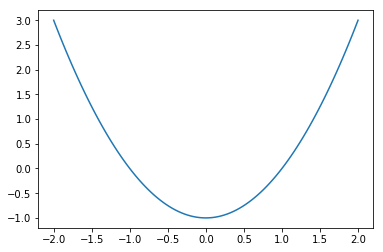Here is a nicer plot:

In :
x = np.linspace(-2, 2, 101)
y = x*x-1
plot3 = plt.plot(x, y,'g')
plt.xlabel('$x$') # The dollar signs denote LaTeX.
plt.ylabel('$y$')
plt.title('The graph of $y=x^2-1$')
plt.grid(True)
plt.show()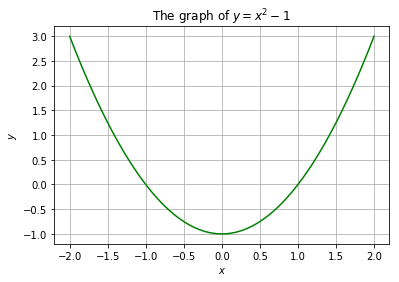The plot command can also plot several functions at once. You just repeat the notation inside of plt.plot() twice. For example:

In :
plt.plot(x, 2*x, 'r', x, 4-2*x**2, 'g')
plt.grid(True)
plt.show()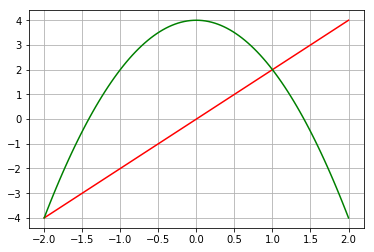There is also no reason that the x-values have to be equally spaced. Here is an example.

In :
theta_values = np.linspace(0, 2*np.pi, 100)
plt.plot(np.cos(theta_values), np.sin(theta_values))
plt.show()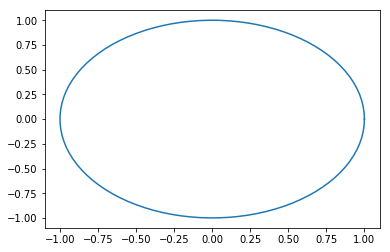Because of the aspect ratio, the circle looks like an ellipse. The following corrects this.

In :
theta_values = np.linspace(0, 2*np.pi, 100)
plt.axes().set_aspect(1)
plt.plot(np.cos(theta_values), np.sin(theta_values))
plt.show()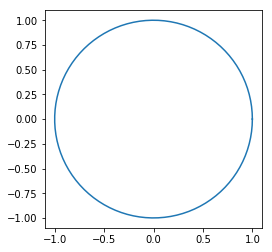## A taste of functions¶

I think it is good to introduce the idea of simple python functions for mathematics. (In programming, there is another related notion of function.)

The following represents the function $f(x)=x^3-x$.

In :
def f(x):
return x**3 - x


The function can act on numbers:

In :
f(1)

Out:
0
In :
f(2)

Out:
6

It also can be used for plotting.

In :
x_values = np.linspace(-1.5, 1.5, 100)

In :
plt.plot(x_values, f(x_values))
plt.show()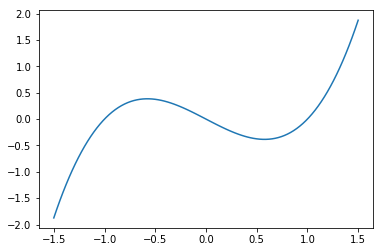Functions don't need to simply return numbers, they can return any Python object. In particular, a function can return a function. Here is an example of currying, when a function returns a function.

The Gaussian with expected value $m$ and variation $s$ is given by the formula $$f(x)=\frac{1}{s \sqrt{2 \pi}} \exp \left(-\frac{1}{2} \left(\frac{x-m}{s}\right)^2\right).$$

In :
from numpy import sqrt, exp, pi

def gaussian(m, s):
def f(x):
return exp(-0.5 * ((x-m)/s)**2)/(sqrt(2*pi)*s)
return f


Note that gaussian returns the function f, and the function f can make use of the variables m and s that were passed to Gaussian.

The following gives the gaussian with $m=0$ and $s=2$.

In :
g = gaussian(0, 2)


Then we can evaluate $g$ like a normal function.

In :
g(1)

Out:
0.17603266338214976

The following plots the Gaussian.

In :
x_values = np.linspace(-10, 10, 100)
plt.plot(x_values, g(x_values))
plt.show()